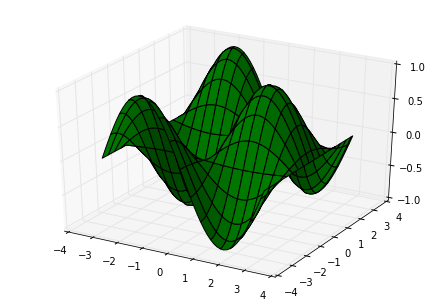# Plotting of Functions of 2 Variables¶

In :
%matplotlib inline
import numpy as np


## Contour plots¶

In :
from math import pi
import matplotlib.pyplot as plt
from numpy import meshgrid, sin, cos, linspace

N = 50
x = linspace(-pi, pi, N)
y = linspace(-pi, pi, N)
X, Y = meshgrid(x,y)
Z = sin(X) * cos(Y)

plt.contour(X, Y, Z)
plt.axis('equal')
plt.show()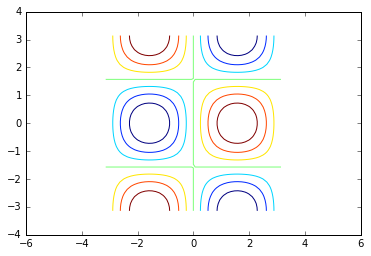In :
from math import pi
import matplotlib.pyplot as plt
from numpy import meshgrid, sin, cos, linspace

N = 50
x = linspace(-pi, pi, N)
y = linspace(-pi, pi, N)
X, Y = meshgrid(x,y)
Z = sin(X) * cos(Y)

plt.contourf(X, Y, Z)
plt.axis('equal')
plt.show()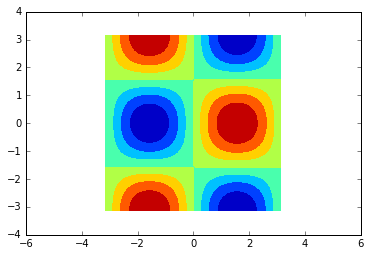## Pseudocolor plots¶

Plot the function $f(x,y)=x^2−y^2$ on the domain $[−2,2]×[−2,2]$.

In :
x, y = np.meshgrid(np.linspace(-2, 2, 100),
np.linspace(-2, 2, 100))

f = x**2 - y**2

In :
plt.figure()
plt.xlim(-2, 2)
plt.ylim(-2, 2)
plt.pcolormesh(x, y, f)
plt.show()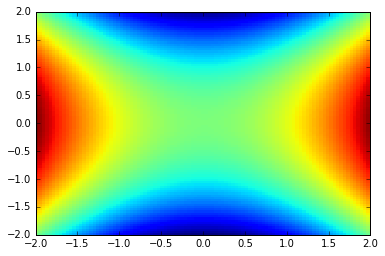This is a good start! However, something about this is asthetically unsatisfying...it doesn't do a good job highlighting an important aspect of the function...the zero set. Let's see how we can make a quick change to improve this.

In :
plt.figure()
plt.xlim(-2, 2)
plt.ylim(-2, 2)
plt.pcolormesh(x, y, f, cmap='RdBu')
plt.show()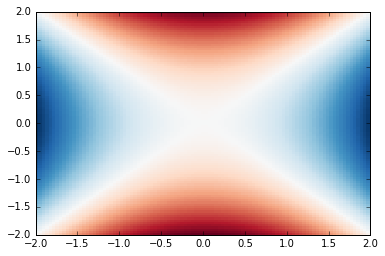As an aside, Matplotlib supports a number of different colormaps. Some of these have particular purposes. There are three general classes called sequential, diverging and qualitative. In this case, we are using the fact the diverging colormaps are good at highlighting a distinct central value in a dataset. If you're interested, you can learn more about this here.

## Wireframe plots¶

In :
from math import pi
import matplotlib.pyplot as plt
import mpl_toolkits.mplot3d as mpl3
from numpy import meshgrid, sin, cos, linspace

N = 50
x = linspace(-pi, pi, N)
y = linspace(-pi, pi, N)
X, Y = meshgrid(x,y)
Z = sin(X) * cos(Y)

fig = plt.figure()
ax = mpl3.Axes3D(fig)
ax.plot_wireframe(X, Y, Z)
plt.show()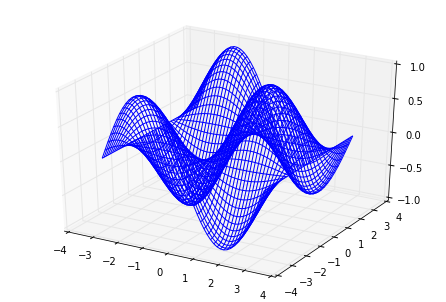## Surface plots¶

In :
from math import pi
import matplotlib.pyplot as plt
import mpl_toolkits.mplot3d as mpl3
from numpy import meshgrid, sin, cos, linspace

N = 50
x = linspace(-pi, pi, N)
y = linspace(-pi, pi, N)
X, Y = meshgrid(x,y)
Z = sin(X) * cos(Y)

fig = plt.figure()
ax = mpl3.Axes3D(fig)
ax.plot_surface(X, Y, Z, rstride=3, cstride=3, color='g')
plt.show()